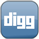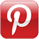### Drawing an ArcOver the weekend, we looked at drawing a curve. We could use this to draw an arc, but there are better ways to do that using the canvas API.

There are two methods you can use to draw an arc, arc() and arcTo(). See below for the method definitions.

``` context.arc(x, y, radius, startingAngle, endingAngle, counterClockwise); context.arcTo(x1, y1, x2, y2, radius); ```

The arc() method takes the center of our circle as the x and y parameters, and the radius of the circle. The starting and ending angles are in radians. The last parameter is a boolean that tells whether the drawing should be clockwise (false) or counter-clockwise (true). To convert degrees to radians, you can simply multiply the degrees by PI divided by 180.

The arcTo() method is a bit different; it draws a line from the current position to x1, y1, and then arcs to x2, y2 with the given radius.

The below draws an arc with a radius of 50, where the starting angle is 20 degrees, and the ending angle is 80 degrees.

``` context.arc(100, 100, 50, 20 * (Math.PI / 180), 80 * (Math.PI / 180), false); ```